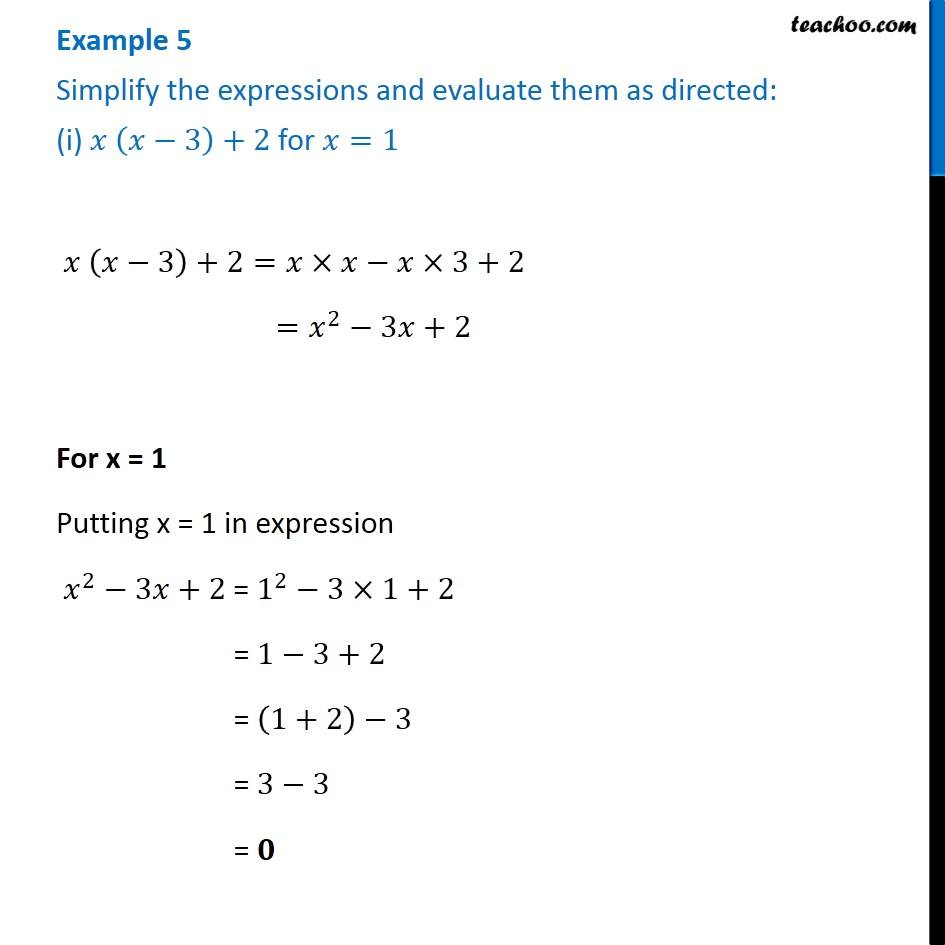Multiplication of Monomials by Binomials and Trinomials

Chapter 8 Class 8 Algebraic Expressions and Identities
Concept wiseLearn in your speed, with individual attention - Teachoo Maths 1-on-1 Class

### Transcript

Example 5 Simplify the expressions and evaluate them as directed: (i) 𝑥 (𝑥−3)+2 for 𝑥=1 𝑥 (𝑥−3)+2=𝑥×𝑥−𝑥×3+2 =𝑥^2−3𝑥+2 For x = 1 Putting x = 1 in expression 𝑥^2−3𝑥+2 = 1^2−3×1+2 = 1−3+2 = (1+2)−3 = 3−3 = 𝟎